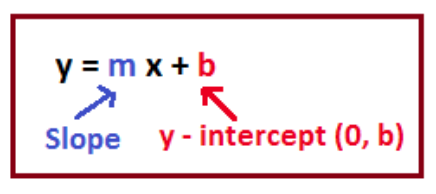# How do you find the slope and intercept of y=3/5x-1?

Aug 3, 2018

Slope: $\frac{3}{5}$

x-intercept: $\left(\frac{5}{3} , 0\right)$

y-intercept: $\left(0 , - 1\right)$

#### Explanation:

$y = \frac{3}{5} x - 1$

This equation is in slope-intercept form:Based on the image, we know that the slope is the value multiplied by $x$, so the slope is $\frac{3}{5}$.

We know the $y$-intercept is $b$, or $- 1$, so it is at $\left(0 , - 1\right)$.

To find the $x$-intercept, plug in $0$ for $y$ and solve for $x$:
$0 = \frac{3}{5} x - 1$

$1 = \frac{3}{5} x$

$\frac{5}{3} = x$

$x = \frac{5}{3}$

The $x$-intercept is at $\left(\frac{5}{3} , 0\right)$.

Hope this helps!

Aug 4, 2018

Slope $\frac{3}{5}$, $y$-intercept $- 1$, $x$-intercept $\frac{5}{3}$

#### Explanation:

The good thing is that this equation is in slope-intercept form

$y = m x + b$, with slope $m$ and a $y$-intercept of $b$.

By pattern matching, we see that our slope is $\frac{3}{5}$, and our $y$-intercept is $- 1$.

We can go about finding our $x$-intercept by setting $y$ equal to zero. We get

$\frac{3}{5} x - 1 = 0 \implies \frac{3}{5} x = 1 \implies x = \frac{5}{3}$

Therefore, our slope is $\frac{3}{5}$, our $y$-intercept is $- 1$, and our $x$-intercept is $\frac{5}{3}$.

Hope this helps!